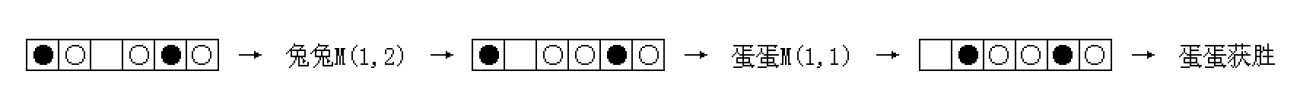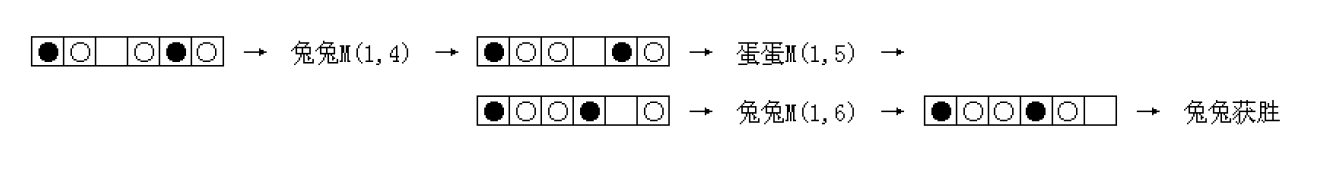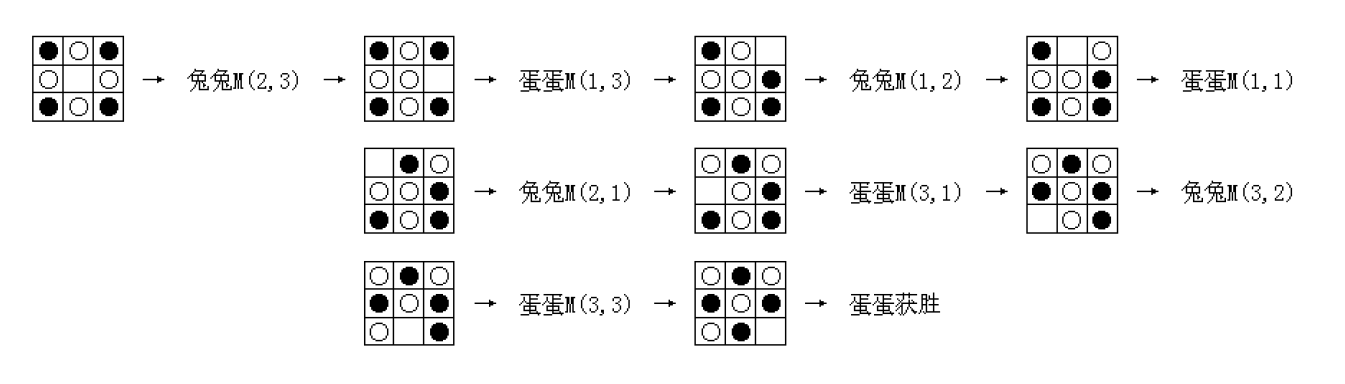/ Vijos / 题库 /

# 描述# 样例1

## 样例输入1

``````1 6
XO.OXO
1
1 2
1 1
``````

## 样例输出1

``````1
1
``````

# 样例2

## 样例输入2

``````3 3
XOX
O.O
XOX
4
2 3
1 3
1 2
1 1
2 1
3 1
3 2
3 3
``````

## 样例输出2

``````0
``````

# 样例3

## 样例输入3

``````4 4
OOXX
OXXO
OO.O
XXXO
2
3 2
2 2
1 2
1 3
``````

## 样例输出3

``````2
1
2
``````

# 提示

1~2: n=1, 1<=m<=20
3: n=3, m=4
4~5: n=4, m=4
6~7: n=4, m=5
8: n=3, m=7
9~14: n=2, 1<=m<=40
15~16: 1<=n<=16, 1<=m<=16
17~20: 1<=n<=40, 1<=m<=40

# 来源

NOI 2011 DAY2 兔兔和蛋蛋的游戏

ID
1718

4

98

43

44%

3

RP++分类题库